xRotational speedEncyclopedia
Rotational speed tells how many complete rotations (i.e. revolutions or cycles) there are per time unit. It is therefore a cyclic frequency
Frequency
Frequency is the number of occurrences of a repeating event per unit time. It is also referred to as temporal frequency.The period is the duration of one cycle in a repeating event, so the period is the reciprocal of the frequency...

, measured in hertz
Hertz
The hertz is the SI unit of frequency defined as the number of cycles per second of a periodic phenomenon. One of its most common uses is the description of the sine wave, particularly those used in radio and audio applications....

(revolutions per second) in the SI System
Si
Si, si, or SI may refer to :- Measurement, mathematics and science :* International System of Units , the modern international standard version of the metric system...

. The units revolutions per minute
Revolutions per minute
Revolutions per minute is a measure of the frequency of a rotation. It annotates the number of full rotations completed in one minute around a fixed axis...

(rpm or 1/min) are more common in everyday life.

Rotational speed can measure, for example, how fast a motor is running. Rotational speed and angular speed are sometimes used as synonyms, but typically they are measured with a different unit. Angular speed, however, tells the change in angle
Angle
In geometry, an angle is the figure formed by two rays sharing a common endpoint, called the vertex of the angle.Angles are usually presumed to be in a Euclidean plane with the circle taken for standard with regard to direction. In fact, an angle is frequently viewed as a measure of an circular arc...

per time unit, which is measured in radians per second
The radian per second is the SI unit of angular velocity, commonly denoted by the Greek letter ω...

in the SI system. Since there are 2π radians per cycle, or 360 degrees per cycle, we can convert angular speed to rotational speed by: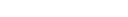and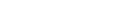where
•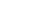is rotational speed in cycles per second
•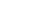is angular speed in radians per second
•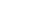is angular speed in degrees per second

For example, a stepper motor
Stepper motor
A stepper motor is a brushless, electric motor that can divide a full rotation into a large number of steps. The motor's position can be controlled precisely without any feedback mechanism , as long as the motor is carefully sized to the application...

might turn exactly one complete revolution each second.
Its angular speed is 360 degrees
Degree (angle)
A degree , usually denoted by ° , is a measurement of plane angle, representing 1⁄360 of a full rotation; one degree is equivalent to π/180 radians...

per second (360°/s), or 2π radian
Radian is the ratio between the length of an arc and its radius. The radian is the standard unit of angular measure, used in many areas of mathematics. The unit was formerly a SI supplementary unit, but this category was abolished in 1995 and the radian is now considered a SI derived unit...

s per second (2π rad/s), while the rotational speed is 60 rpm.

Rotational speed is not to be confused with tangential speed, despite some relation between the two concepts. Imagine a rotating merry-go-round. No matter how close or far you stand from the axis of rotation, your rotational speed will remain constant. However, your tangential speed does not remain constant. If you stand two meters from the axis of rotation, your tangential speed will be double the amount if you were standing only one meter from the axis of rotation.